# How To Understand and Calculate Sports Odds

Home / Blog / Betting Tips / How To Understand and Calculate Sports Odds

Posted on 03 Sep 2021 | Category: Betting Tips | Author:BetAdrian

## Understanding sports odds: what are they?

If you are willing to place a bet on the upcoming match but lack the know-how to do so, then we have got you covered. We will help you in understanding sport betting in-depth.

Firstly, you have to make out what odds mean and which bets are worth the risk or not. You'll come to know about all this in the sections below.

Secondly, you need to apprise yourself about the diverse formats in which odds are depicted. The different formats used for the odds are as follows:

• American ( plus/minus )
• Decimal odds
• Fractional odds

It's quite easy to learn each of these formats, and we'll show you this in the upcoming sections below.

Lastly, with the help of odds, you can calculate the implied probability of a given outcome in sporting events.

After understanding how do odds work, you'll easily come to know the best offering you the best payouts and the money you need to wager.

## What are American, decimal, and fractional odds?

Usually, the sportsbooks will display the odds either in American, decimal, or fractional formats. Moreover, the odds serve two purposes:

1. At first, they depict the implied probability of an outcome to which they are attached.

2. Secondly, they also indicate the amount of money you could win by betting on that outcome.

But one should not rely completely on odds only as there are chances of an event occurring, especially when evaluating the probability. Some factors influence the odds more than anything else.

Some sportsbooks try their best to manipulate the odds to incentivize the bets on a certain side. As the sportsbook takes a cut baked directly onto the odds, the sum of probabilities for a single event will always surpass 100 percent.

By looking at odds, you will also come to know how much the bookmakers charge for your bet.

## How to read American odds in sports betting?

If you are also wondering- how to read sports odds, you have come to the right place. The plus and minus signs on the sports betting line indicate whether the bet is placed on the favorite or underdog.

The negative numbers on the betting line indicate the favorite. In other words, how much one needs to bet to win the odds also depicts a certain amount.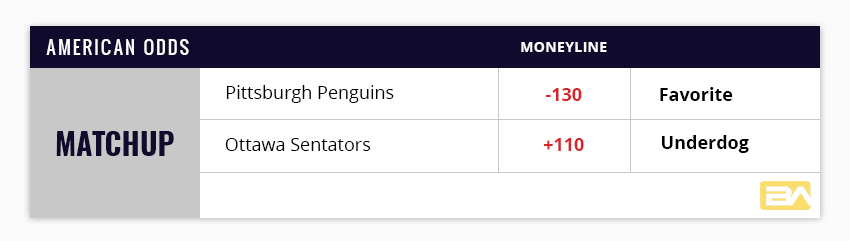The positive number indicates betting on the underdog. Along with this, it will also signify the amount of money you can win if you bet a certain amount.

Usually, all the popular wagers, including the point spread bets, will witness both sides with negative odds. But when you are betting on the spread, you need to risk more than you profit as the point spread levels the playing field between two sides. Understanding sport betting odds will become easy for you this way.

## How to calculate my bet with American odds

Using the formula mentioned below, you can very easily calculate the potential winnings for any value you want.

If you go about placing a \$40 bet on Pittsburgh (-130), your equation will seem like this:• You need to cross multiply, and you will get 130x=4000. Then find the value of x.
• x= 4000/130
• x= 30.77

It means you will win \$30.77 if you bet \$40 on Pittsburgh. Consequently, the payout will be \$70.77, which will comprise your original bet plus your winnings.

## Calculating implied probability with American odds

The implied probability is suggested by the odds and refers to the possibility of a certain outcome. If you need to figure it out, then convert the odds to the percentage, and this percentage will tell you whether the event will happen or the alternative.

Implied probability is useful to find the estimate of an event. If your estimate comes out to be different from the sportsbooks, you should adjust your bet accordingly.

If one team has a 60% chance of winning and its implied probability is 52.4%, it will be a smart bet.

The implied probability of the outcomes of the game is usually above 100%. In betting terms, it is known as overground. If you convert the odds to a percentage, then it will also include the vig. Above all, if you want a more accurate picture of what oddsmakers expect in the game, it is necessary to remove the vig from the betting lines.

If you want to calculate the implied probability, then you can make use of this formula:

Risk/Return = implied probability

Ever wondered what this would look like with American odds. Let us start with positive odds, as they are the easiest to work with.

### You can use this formula with positive odds: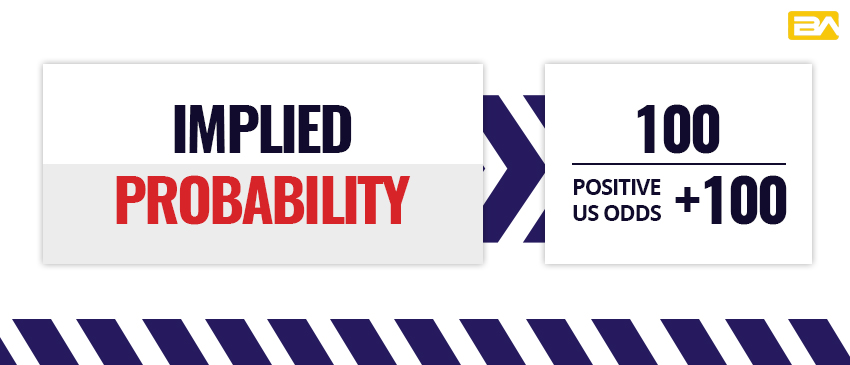If you want to calculate the implied probability of winning the game in Ottava's case, you can do so by using the same formula. But you cannot use this formula for Pittsburgh because they possess negative odds. Let us witness Ottava's odds by putting it into the formula:

So, according to the bookmaker, Ottava has a 47.6% chance of winning. One should place this bet if one thinks their chances of winning are more.

## How do negative odds differ?

One should not get confused by all the brackets and parentheses. Their sole purpose is to flip the sign on the negative US odds. Here's the formula for the same: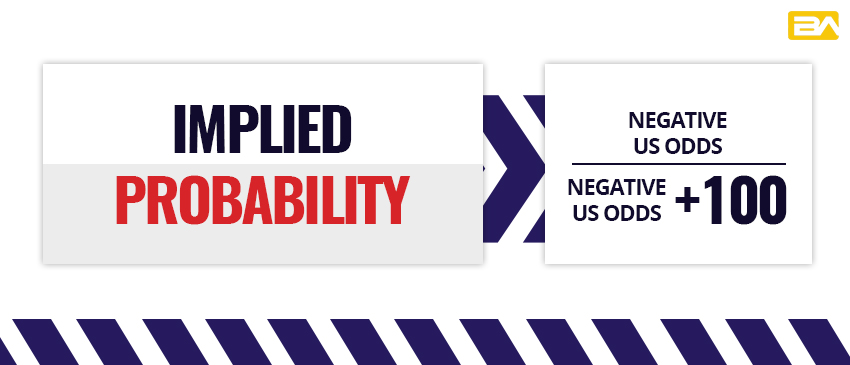It gets even simpler when you tend to use actual numbers. Using the above example, you can calculate the Pittsburgh implied probability of winning the game. But as Ottava has positive odds, you cannot use this formula for this.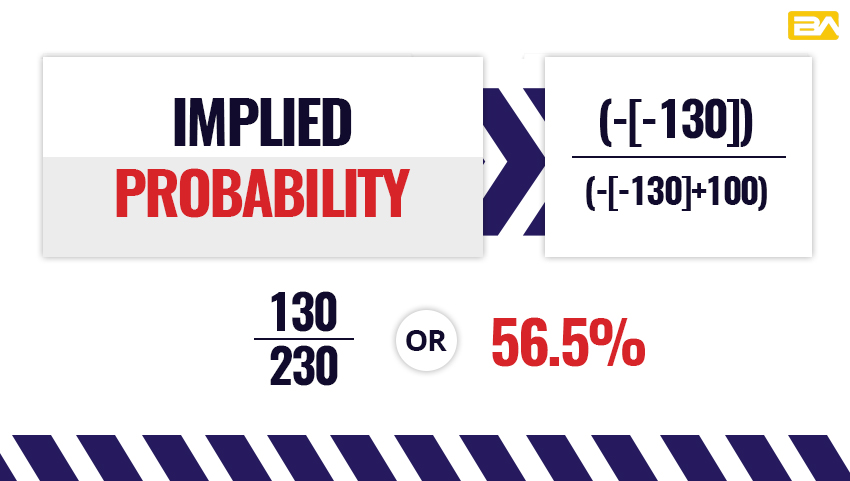According to the bookmaker, Pittsburgh has a 56.5% chance of winning the game.

### Decimal odds in sports betting explained.

Without any doubt, decimal odds are the most popular type of odds around the world. These are advantageous as compared to the American odds in two terms. It is much easier to convert these to probabilities. Once you become familiar with these, they become more intuitive.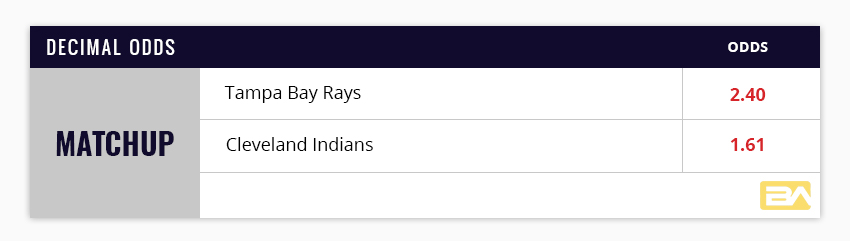### Calculating winnings with decimal odds

You can very easily determine your payout with the decimal odds. You simply need to multiply your wager by the odds concerning the team on which you are betting. You need to subtract the original wager from the payout to calculate the winnings. Let us have a look: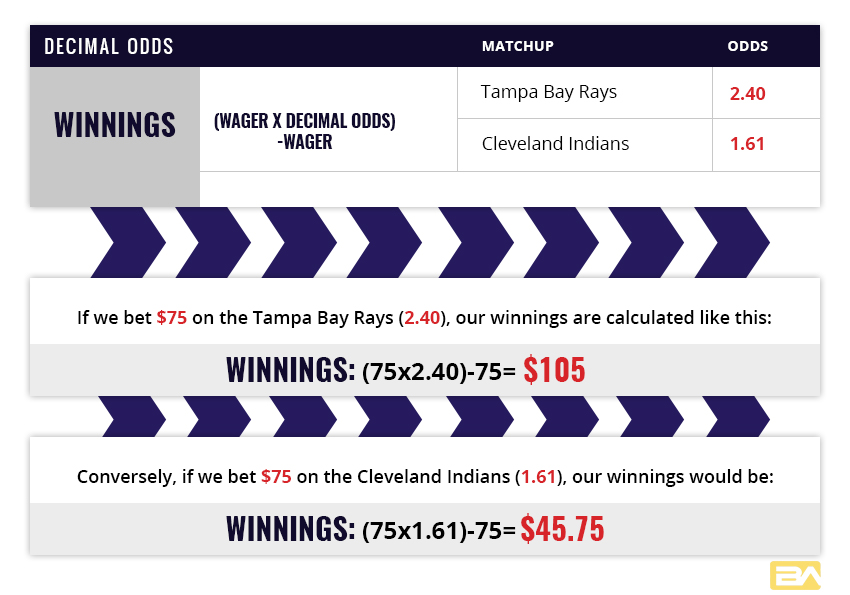### Calculating implied probability

By calculating the implied probability, you can determine if the wager is worth the risk or not. Using the following formula, you can make out this:

## How do you understand odds in sports betting with fractional odds?

Some bettors like to bet on a horse in the Breeder's cup classic.

Bettors open up their app and see strange-looking figures like 8/3 or ½. In this situation, how can you calculate the odds? It is pretty simple if you ask us.

The number on the left side indicates how many times the oddsmaker expects the related outcome to fail. Contrary to it, the number on the right side indicates the number of times the outcome should succeed.

## Ever wondered what this means for your pocketbook?

### Calculating payouts

If it is fractional betting, then calculating your payout is quite easy. You need to multiply your bet with the numerator and then divide the number by the denominator.

For instance, if you go about placing a \$50 bet on 8/3 odds, the calculation will look like this:

(50 x 8) / 3 = \$133.33 ( a total of \$183.33 coming your way ).

But if you place a \$50 bet on the ½ odds, then the calculation will be as follows:

( 50 x 1 ) / 2 = \$ 25 ( a total of \$75 in your pocket.

### Calculating implied probability

It is much easier to figure out the implied probability for the fractional bets. For instance, your choice of the horse has 8/3 odds on him. It means that your horse is expected to win eight times out of 11 races.

You will get your implied probability by dividing the number of times he is expected to win by the number of races on the roster. The implied probability for the same will be 8/11 = 72.7%.

But always keep one thing in mind that whenever you are calculating the odds, be it American, decimal, or fractional, it will always come out to be more than 100% when you add together the outcomes. This is what we term as vig or the cut.

### What is push?

Every bettor needs to learn about the concept known as a push along with the odds in sports betting. A push can directly affect your payout, but more than that, push only happens when there is a tie.

Some games allow the final games to be a tie. If this is the case, then you can bet on a tie as the outcome. But some people mistake push to be betting on the Moneyline, but it is not so. Your whole money will be refunded when a tie happens.

You can easily be successful with sports betting as it is a unique form of paid entertainment. But if you want this to happen, try to familiarize yourself with the betting odds and their kinds.

If you place more informed bets, then you'll have an insight into your chances of winning. Consequently, you'll have a betting chance of winning money from the bet.

You can take the help of the calculators for the betting odds given in the sportsbook. Doing so will ensure that you have calculated it accurately. Besides this, it will help you in understanding sports betting by practicing this.

### Ready to find some favorable odds?

Now, you must have enough information to become an expert in reading sports odds, so get out and win some money. Usually, the informed bettors are the sharp bettors as not all sports are created equal. If you want to know how to bet on sports before laying down your first wager, then check out our comprehensive guide for the same.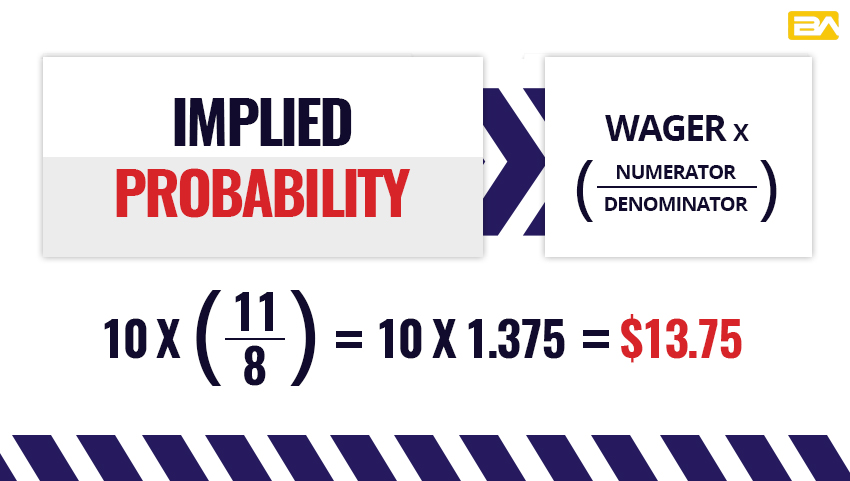When betting on Alvarez, the underdog, the potential winnings will be higher. But keep this thing in mind that there is a reason that they are known as underdogs, be its rookie season, recent injury, or serious competition. If you bet on the underdogs, it means less risk, which will pave the way for you to win more money.

But contrary to it, if you bet on the favorite, Golovkin, you need to risk more to get the same winnings. This way, it will be easy for you understanding sport betting odds.

#### What is The Over/Under in Sports Betting?

BetAdrian has been providing odds to sports fans all around the world since 2007. We are the only online sportsbook dedicated to sports betting that combines European and American styles. Our objective is to make online sports betting as simple, easy, secure, and enjoyable as possible. Along with that, we'll publish the best online sports betting tips and techniques, betting tactics, industry news, strategies, and much more.

0 Response(s)